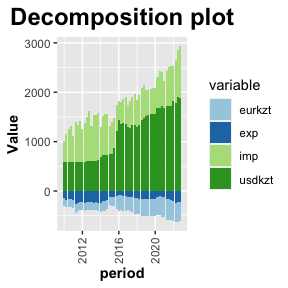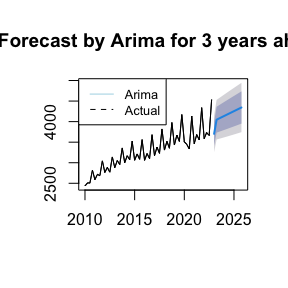# Regression-model

## Introduction

As regressors are chosen for a linear regression model, AFR package recommends to check for:

### 1. Optimal size of the time-series data

Function opt_size assess whether time-series data has enough observations for the chosen model.

model<-lm(real_gdp~imp+exp+usdkzt+eurkzt, macroKZ)
opt_size(model)
#> There is acceptable number of observations.
#> It is necessary to have 24 observations.
#> Your regression has 52 observations.
#> Warning in opt_size(model): If there is equal or close number of observations,
#> please check further.

Based on the output of the function, modify the model, i.e. remove or add regressor(s).

### 2. Choose the best regression model

From the initially built linear regression model regsel_f function allows to choose the best regressors by Akaike Information criterion (AIC) and Adjusted R-squared (Adj R2) parameters. These parameters are set by default, but other parameters can be added too.

To dive into details, check_betas function demonstrates all models with regressors’ betas based on which regsel_f function gives the result. A user can export the output of all models into Excel document for more representative format by using function write_xlsx of writexl package.

check_betas(model)

### 3. Analysis of the model

As regsel_f gave the best regression model, it can be analysed by diagnostic tests for the compliance with Gauss-Markov theorem for a multiple regression model.

Graphically, the regression model can be visualized for decomposition and forecasting. Function dec_plot demonstrates a contribution of each regressor in a form of stacked bar plot.

dec_plot(model, macroKZ)Function reg_plot shows actual and forecast data. Forecasting can be performed by Arima or trending.

reg_plot(model, macroKZ)